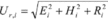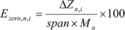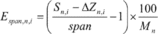# Title 43

## SECTION 3175.135

### 3175.135 Uncertainty determination.

§ 3175.135 Uncertainty determination.

(a) Reference uncertainty calculations for each transducer of a given make, model, URL, and turndown must be determined as follows (the result for each transducer is denoted by the subscript i):

(1) Maximum error (Ei). The maximum error for each transducer is the maximum difference between any input value from the test device and the corresponding output from the transducer under test for any required test point, and must be expressed in percent of transducer span.

(2) Hysteresis (Hi). The testing required in § 3175.132 requires at least three pairs of tests using both ascending test points (low to high) and descending test points (high to low) of the same value. Hysteresis is the maximum difference between the ascending value and the descending value for any single input test value of a test pair. Hysteresis must be expressed in percent of span.

(3) Repeatability (Ri). The testing required under § 3175.132 requires at least three pairs of tests using both ascending test points (low to high) and descending test points (high to low) of the same value. Repeatability is the maximum difference between the value of any of the three ascending test points for a given input value or of the three descending test points for a given value. Repeatability must be expressed in percent of span.

(b) Reference uncertainty of a transducer. The reference uncertainty of each transducer of a given make, model, URL, and turndown (Ur,i) must be determined as follows:Where Ei, Hi, and Ri, are described in paragraph (a) of this section. Reference uncertainty is expressed in percent of span.

(c) Reference uncertainty for the make, model, URL, and turndown of a transducer (Ur) must be determined as follows:

Ur = s × tdist Where: s = the standard deviation of the reference uncertainties determined for each transducer (Ur,i) tdist = the “t-distribution” constant as a function of degrees of freedom (n-1) and at a 95 percent confidence level, where n = the number of transducers of a specific make, model, URL, and turndown tested (minimum of 5)

(d) Influence effects. The uncertainty from each influence effect required to be tested under § 3175.133 must be determined as follows:

(1) Zero-based errors of each transducer. Zero-based errors from each influence test must be determined as follows:Where: subscript i represents the results for each transducer tested of a given make, model, URL, and turndown subscript n represents the results for each influence effect test required under § 3175.133 Ezero,n,i = Zero-based error for influence effect n, for transducer i, in percent of span per increment of influence effect Mn = the magnitude of influence effect n (e.g., 1,000 psi for static-pressure effects, 50 °F for ambient temperature effects) And: DZn,i = Zn,i−Zref ,i Where: Zn,i = the average output from transducer i with zero input from the test device, during the testing of influence effect n Zref,i = the average output from transducer i with zero input from the test device, during reference testing.

(2) Span-based errors of each transducer. Span-based errors from each influence effect must be determined as follows:Where: Espan,n,i = Span-based error for influence effect n, for transducer i, in percent of reading per increment of influence effect Sn,i = the average output from transducer i, with full span applied from the test device, during the testing for influence effect n.

(3) Zero- and span-based errors due to influence effects for a make, model, URL, and turndown of a transducer must be determined as follows:

Ez,n = sz,n × tdist Es,n = ss,n × tdist Where: Ez,n = the zero-based error for a make, model, URL, and turndown of transducer, for influence effect n, in percent of span per unit of magnitude for the influence effect Es,n = the span-based error for a make, model, URL, and turndown of transducer, for influence effect n, in percent of reading per unit of magnitude for the influence effect sz,n = the standard deviation of the zero-based differences from the influence effect tests under § 3175.133 and the reference uncertainty tests, in percent ss,n = the standard deviation of the span-based differences from the influence effect tests under § 3175.133 and the reference uncertainty tests, in percent tdist = the “t-distribution” constant as a function of degrees of freedom (n-1) and at a 95 percent confidence level, where n = the number of transducers of a specific make, model, URL, and turndown tested (minimum of 5).